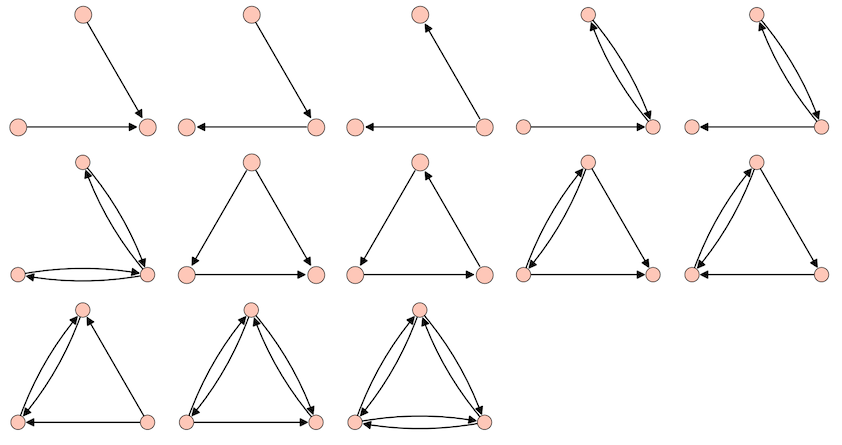# Digraphs database

I'm interested in the list of digraphs without muliedges and I used the following command, but it does not give me back any output.

for G in digraphs(3,lambda G: G.allow_multiple_edges(False)):
print(G.show())


I'm wondering why there is no output and how I can fix it.

edit retag close merge delete

G.allow_multiple_edges is returning nothing, but acting on the graph's options

The digraphs returned by the digraphs generator are simple (multiple edges not allowed).

If you want digraphs without symmetric pairs, you can do:

sage: for g in digraphs(3):
....:     if any(g.has_edge(v, u) for u, v in g.edge_iterator(labels=False)):
....:         continue
....:     print(g.edges(labels=False))


Sort by » oldest newest most voted

To get all the connected directed graphs on 3 vertices (no multiple directed edges, no loops) up to isomorphism, one can use graphs.nauty_geng and digraphs.nauty_directg as follows:

sage: n = 3

sage: graphs_n = [g for g in graphs.nauty_geng(f"-c {n}")]
sage: digraphs_n = list(digraphs.nauty_directg(graphs_n))

sage: len(digraphs_n)
13

sage: opt = dict(layout='circular', vertex_size=600, vertex_labels=False)
sage: opt = dict(xmin=-1, xmax=1, ymin=-0.7, ymax=1.1, **opt)
sage: G = graphics_array([g.plot(**opt) for g in digraphs_n], ncols=5)

sage: G.show(figsize=(17, 9))
Launched png viewer for Graphics Array of size 3 x 5more